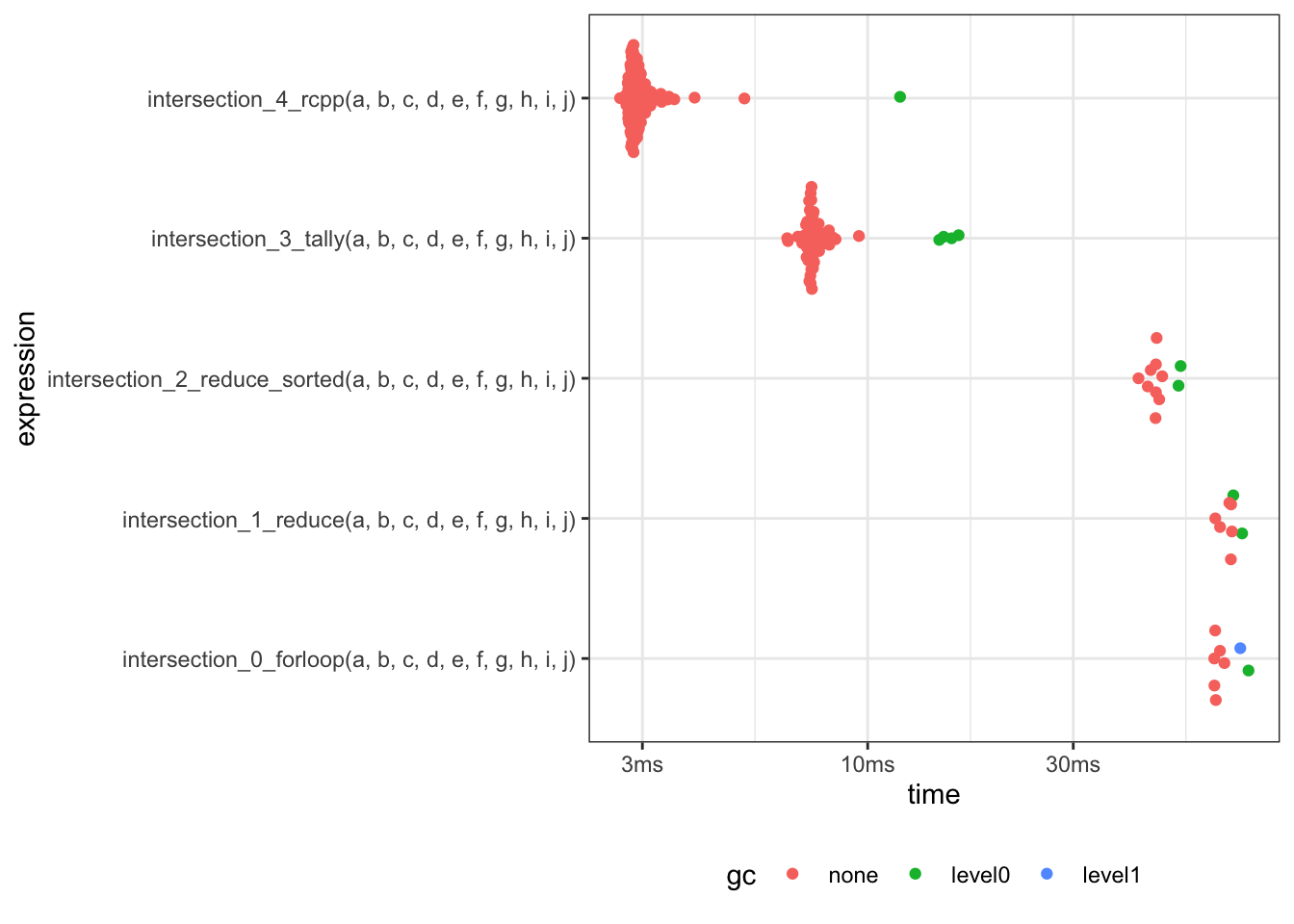# Intersection of multiple vectors

## Problem - Finding the intersection of multiple integer vectors

• I want to find the intersection of multiple integer vectors
• Unbounded number of vectors, but usually 4-10
• Unbounded size, but usually 10 to a million elements
• Duplicated elements allowed in output
• Order not important
• Submissions welcomed! (including alternate test sets that demonstrate a different dominant solution)

## Test set

• Benchmarking data:
• 10 vectors
• Varying lengths
• Elements sampled from range (1, 1e5)
• I realise that this benchmarking data ignores the following cases
• a few short vectors
• very long vectors
• vectors already sorted by size
• vectors with large overlap
• vectors with no overlap
``````set.seed(1)

source <- seq.int(1e5)
a <- sample(source, size=1.0e5, replace=TRUE)
b <- sample(source, size=0.9e5, replace=TRUE)
c <- sample(source, size=0.8e5, replace=TRUE)
d <- sample(source, size=0.7e5, replace=TRUE)
e <- sample(source, size=0.6e5, replace=TRUE)
f <- sample(source, size=0.5e5, replace=TRUE)
g <- sample(source, size=0.4e5, replace=TRUE)
h <- sample(source, size=0.3e5, replace=TRUE)
i <- sample(source, size=0.2e5, replace=TRUE)
j <- sample(source, size=0.1e5, replace=TRUE)``````

## `for` loop

``````intersection_0_forloop <- function(...) {
ll <- list(...)
working_set <- ll[]
for (l in ll[-1]) {
working_set <- intersect(working_set, l)
}

working_set
}``````
``sort(unique(intersection_0_forloop(a, b, c, d, e, f, g, h, i)))``
``````   1258  4071  5370  7151  7749 14098 20244 23662 28383 32717 32730 40064
 40376 44214 49955 53971 54336 54986 59242 61623 63523 67816 71673 82711
 82976 83606 86895 90685 91729 92002 95154``````

## `Reduce()`

• Acculmulate `intersect()` vector by vector using `Reduce()`
• Under the hood, this works identical to the `for` loop example above…
• … but it’s much better looking :)
``````intersection_1_reduce <- function(...) {
Reduce(intersect, list(...))
}``````
``sort(unique(intersection_1_reduce(a, b, c, d, e, f, g, h, i)))``
``````   1258  4071  5370  7151  7749 14098 20244 23662 28383 32717 32730 40064
 40376 44214 49955 53971 54336 54986 59242 61623 63523 67816 71673 82711
 82976 83606 86895 90685 91729 92002 95154``````

## Sort by length and then `Reduce()`

• Acculmulate `intersect()` vector by vector using `Reduce()`
• By ensuring that the intermediate result is always as short as possible, it should reduce the memory allocations.
``````intersection_2_reduce_sorted <- function(...) {
ll   <- list(...)
lens <- vapply(ll, length, integer(1))
ll   <- ll[order(lens)]
Reduce(intersect, ll)
}``````
``sort(unique(intersection_2_reduce_sorted(a, b, c, d, e, f, g, h, i)))``
``````   1258  4071  5370  7151  7749 14098 20244 23662 28383 32717 32730 40064
 40376 44214 49955 53971 54336 54986 59242 61623 63523 67816 71673 82711
 82976 83606 86895 90685 91729 92002 95154``````

## Tally vector

• Initialise a `tally` vector wtih length equal to the maximum integer across all the vectors.
• For each element in each vector, increase the `tally` for that element by 1.
• Find all counts in the `tally` vector which match the number of vectors given.
• This will be increasingly inefficient as `maxval` gets larger and larger.
``````intersection_3_tally <- function(...) {
ll     <- list(...)
maxval <- max(vapply(ll, max, integer(1)))
tally  <- integer(maxval)
for (elements in ll) {
tally[elements] <- tally[elements] + 1L
}
which(tally == length(ll))
}``````
``sort(unique(intersection_3_tally(a, b, c, d, e, f, g, h, i)))``
``````   1258  4071  5370  7151  7749 14098 20244 23662 28383 32717 32730 40064
 40376 44214 49955 53971 54336 54986 59242 61623 63523 67816 71673 82711
 82976 83606 86895 90685 91729 92002 95154``````

## Rcpp function

• An Rcpp integer-only version of `intersect()`
• An R wrapper to go with it.
• side effect: original vectors are sorted numerically.
``````Rcpp::cppFunction(
"std::vector<int> intersection_rcpp_core(IntegerVector v1, IntegerVector v2) {
std::sort(v1.begin(), v1.end());
std::sort(v2.begin(), v2.end());
std::vector<int> v_intersection;

std::set_intersection(v1.begin(), v1.end(),
v2.begin(), v2.end(),
std::back_inserter(v_intersection));

return v_intersection;
}
")

intersection_4_rcpp <- function(...) {
Reduce(intersection_rcpp_core, list(...))
}``````
``sort(unique(intersection_4_rcpp(a, b, c, d, e, f, g, h, i)))``
``````   1258  4071  5370  7151  7749 14098 20244 23662 28383 32717 32730 40064
 40376 44214 49955 53971 54336 54986 59242 61623 63523 67816 71673 82711
 82976 83606 86895 90685 91729 92002 95154``````

## Benchmark

``````bm <- bench::mark(
intersection_0_forloop      (a, b, c, d, e, f, g, h, i, j),
intersection_1_reduce       (a, b, c, d, e, f, g, h, i, j),
intersection_2_reduce_sorted(a, b, c, d, e, f, g, h, i, j),
intersection_3_tally        (a, b, c, d, e, f, g, h, i, j),
intersection_4_rcpp         (a, b, c, d, e, f, g, h, i, j),
check = FALSE
)``````
expression median itr/sec mem_alloc
intersection_0_forloop(a, b, c, d, e, f, g, h, i, j) 64.17ms 15.42914 10.55MB
intersection_1_reduce(a, b, c, d, e, f, g, h, i, j) 69.37ms 14.69209 10.56MB
intersection_2_reduce_sorted(a, b, c, d, e, f, g, h, i, j) 46.66ms 21.68236 8.24MB
intersection_3_tally(a, b, c, d, e, f, g, h, i, j) 7.41ms 133.20700 3.24MB
intersection_4_rcpp(a, b, c, d, e, f, g, h, i, j) 2.89ms 339.86518 356.72KB## Summary

• Under-the-hood, the `for` loop and the `Reduce()` methods are identical and this is evidenced by their identical runtimes.
• Sorting input vectors by length results in a 2x speed-up over the base case for this benchmark example (which is pretty pathological with the vectors in order of decreasing size)
• Using a tally vector instead of the builtin `intersect()` results in a ~9x speedup over the base case.
• `Rcpp` is clearly the fastest with least memory use.
• This could be extended to be a pure `Rcpp` function with the functionality of the `Reduce()` done in `C++`
• Could also implement the tally/memory technique in `Rcpp`
• Contributions welcomed!